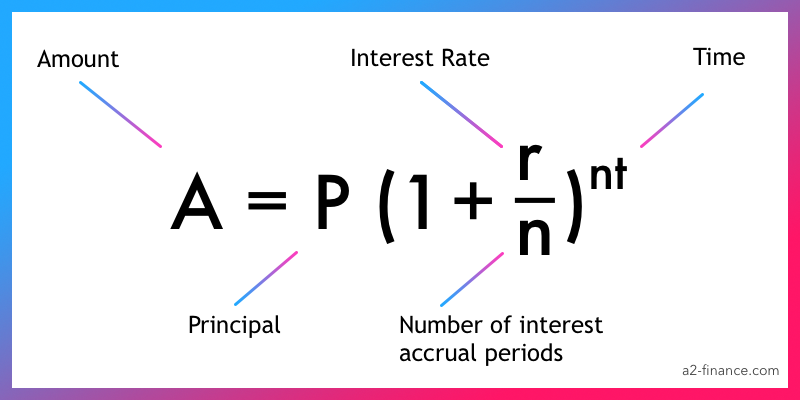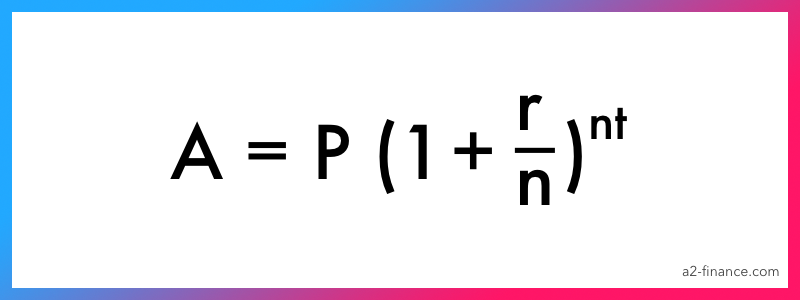Compound Interest Calculator (+ formula and examples)

# Compound Interest Calculator

Step 2 Contribute
$Step 3 Interest accrual % $$\text{Amount} = P \left(1 + {r \over n}\right)^ {nt}$$ Final Balance$0

$0 Total Contributed$0

Total Interest

When considering where to invest money, people, as a rule, only focus on the interest rate. This is logical, since the higher the interest rate, the higher the return on the investment. But there is another factor which influences the net result – the type of interest. In practice, “simple” interest is most commonly found. “Compound” interest is found more rarely, but it generates a bigger profit for the same time period.

## What is compound interest?

Compound interest is the accrual of interest on both the initial investment amount and on the interest earned over the previous years of the investment, ie. interest on interest. For example, after making a deposit, interest for the first year is accrued on the initial amount invested. In the second year, interest is accrued on the initial amount deposited and on the interest received in the previous year. In the third year, interest is accrued on the initial deposit and all the interest previously received.

So, every year, the deposit increases more than it did the previous years. For example, if the deposit grew by $100 2 years ago, then last year it could have increased by$110 and this year by $150. With compound interest, the amount of interest received is not the same year on year. ## How to use a compound interest calculator ### Step 1. Enter the initial investment amount This is the amount which you would like to use when you open an account or with which you would like to begin investing. It is often said that this is the most important parameter, but in practice that is not always the case. Sometimes it is a minor factor. For example, when there is a high interest rate or if the interest rate is paid out frequently. ### Step 2. Enter the amount you will regularly invest Here you should type in the amount which you will periodically add to the initial investment. When you open an account, some banks allow you to add funds at different intervals. This is why this field is essential. If you have decided that you do not wish to add funds to the account, then enter 0. ### Step 3. Type in the interest rate and accrual period This is one of the most important parameters. Enter the interest which will be accrued for each given period. This figure will affect how much you can earn over the long term. ### Step 4. Enter the number of years This is the number of years over which your investment account will be open. It is precisely this figure which is the most important in calculating compound interest. The longer your investment account will be open, the more you can earn, because interest will compound every year. ### Step 5. Press the "Calculate" button By clicking on the "Calculate" button now, you will obtain a detailed chart and formula, explaining how exactly the calculation is worked out (you can only find this kind of graph on our site), and also a table showing the estimated interest earned every year. ## How does compound interest work? Compound interest means the interest accrues on the initial deposit amount, as well as on the earnings received during previous periods. An example of this is a deposit in a bank, where the profit is capitalized. The amount of interest earned the first year on the deposit is added to the initial deposit. So the interest rate is applied to the increased deposit amount in the second year, which guarantees the growth of the resulting profits. ### Compound interest formula Expected earnings from compound interest interest can be calculated using the following formula: A = P x (1 + r/n)nt, where: A = the amount which you will receive at the end of the period, P = the amount of the initial investment, i.e. what you have invested, r = the yearly interest rate, n = the number of interest accrual periods (monthly, every quarter, yearly and so on), t = the overall investment period in years.### Examples of the calculation As we have already established, when compound interest is used, the profit from the investment steadily increases. Now let's look at an example to see how it works. Suppose that you opened an investment account with$1000 for 1 year with an interest rate of 5%. In this case, the earnings at the end of the year will be: $1000 +$1000 * (5 / 100) = $1050. In other words, we simply calculated the amount of simple interest earned over a year. Then we decided that we wanted to keep the investment account open over another year. Therefore we took the$1050 we calculated earlier and again added it to the initial deposit with a 5% interest rate. So it works out as: $1050 +$1050 * (5 / 100) = $1102.5. As you can see, the deposit rises to$1050 at the end of the first year and $1102 the second year. This is an example of the effects of compound interest, i.e. when we use the amount of the initial deposit plus the interest received in the first year (that is,$1050) to work out the interest for the second year and the result is $1102. If we used simple interest, then we would not include the interest earned in the first year plus the deposit ($1050), but we would only use the initial deposit of $1000. This works out as: • 1 year:$1050
• 2 years: $1050 • 3 years:$1050
• 4 years: $1050 • 5 years:$1050

After 5 years, your deposit will have risen to $5250. And this is how your deposit will grow if you use compound interest: • 1 year:$1050
• 2 years: $1102 • 3 years:$1157
• 4 years: $1215 • 5 years:$1276

So after 5 years, your deposit will have increased to \$5800. As you can see, the difference in savings is precisely in the magic of compound interest. Since both the initial deposit and the interest earned in previous years are used to calculate the interest, your earnings will be higher than with simple interest.

### Difference between simple and compound interest

The main difference between these two types of interest is on what exactly interest is earned. When simple interest is used, interest is mainly accrued on the initial amount of money deposited. It makes no difference if you are calculating the amount of interest for the first year or the third – the amount of interest will always be the same. In compound interest, interest is earned on the amount of the initial deposit plus the interest accrued in previous years. In other words, the increased deposit amount after adding the interest earned over the past year is taken as the foundation for the calculation of the current year's interest. In brief, the basis for simple interest is always the same. For compound interest, it is always different.

 Simple interest Compound interest Interest is accrued once – at the end of the period Interest is accrued every year Only the initial deposit is taken into account in the calculation The initial deposit plus the yearly earnings are taken into account in the calculation The profit will be the same every year The profit will increase every year, i.e. it will differ

### How to calculate compound interest?

Calculate how much you earn on your investment for one year. Then take this amount to calculate the interest rate, rather than the initial investment amount. In other words, use the same interest rate, but different amounts. Start with the first year, then the second and so on.

Here is the general formula if interest is accrued on a yearly basis: P x (1 + r)t, where P – is your initial deposit, r – is the yearly interest rate and t – is the number of years.### When do you need to use compound interest?

If you put some money aside, for example, by making a deposit into a bank account, compound interest can help you to find out how much interest you will receive at the end of the investment.

If you take out a loan, then compound interest can help you to find out how much you will owe at the end of the loan period.

### What is the formula for compound interest?

The usual formula looks like this: P x (1 + r/n)nt, where P is the initial investment amount, r is the interest rate, n is the number of interest accrual periods and t is the overall duration of the investment in years.

### Why is compound interest preferable to simple interest?

The main reason for this is that, when you use compound interest, you earn more money at the end of the investment period than when using simple interest. This is the main advantage.

### What factors affect the calculation of compound interest?

There are four of them:

• the initial investment amount, i.e. how much you deposit or open the account with;
• the additional deposits, i.e., the amount which you deposit into the account on a regular basis and the frequency of deposits;
• the interest rate, i.e., the percentage accrued each year for the duration of the investment;
• the duration of the investment, i.e., the period during which the investment account is open (most often in years, but sometimes in months or even days).